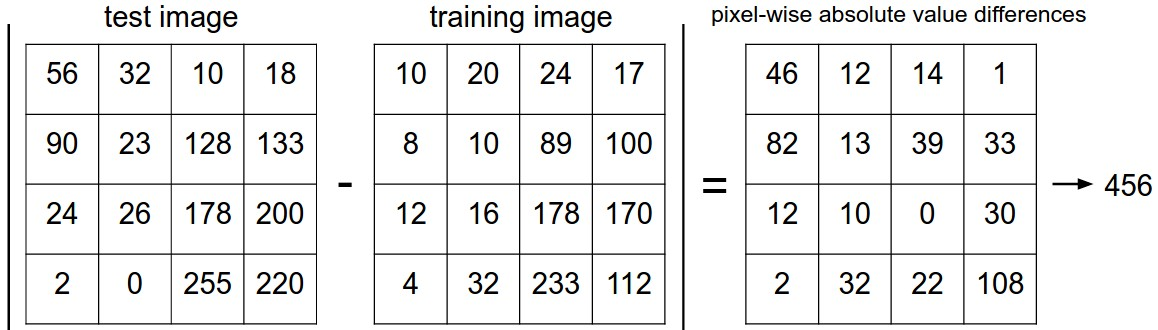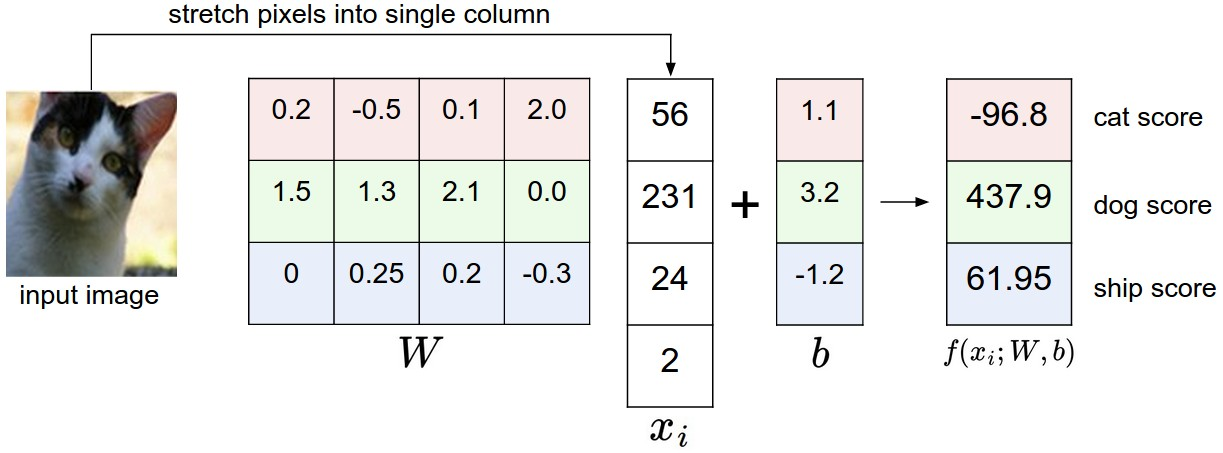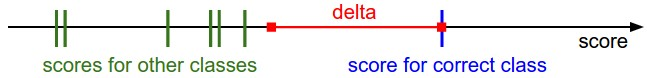CS231n 回顾与思考(一)Xin Qiu Feb 10, 2017
•## 图像分类

### 最近邻分类器

$$d_{1} (I_{1}, I_{2})=\sum_{p} \left| I^p_1 - I^p_2 \right|$$$$d_{1} (I_{1}, I_{2})= \sqrt{\sum_{p} \left( I^p_1 - I^p_2 \right)^2}$$

### KNN

KNN 思路是找出最相似的 k 个图片的标签，然后取这 k 个中相同标签最多的那个标签。上面的 NN 其实就是 k = 1 的情况。

### 线性分类器

$$f(x_i, W, b) = W x_i + b$$### 多类支持向量机损失

SVM的损失函数是在所有正确分类的得分和不正确分类的得分中确定出一个边界差 $\Delta$。之前提到过，第 $i$ 个数据包含图像 $x_i$ 的像素和代表正确标签的 $y_i$。就数据集 CIFAR-10 而言，类别有10种，所以 $f(x_i, W, b)$ 计算能得出对于10个类的分别得分，正确的是在类 $i$ ，错误的类标签就是 $j$。那么对于第$j$类的得分就是第$j$个元素：$s_j = f(x_i, W)_j$。根据概念，可以得出 SVM 的损失函数

$$L_{i} = \sum_{j\neq y_{i}} \max(0, s_{j} - s_{y_{i}} + \Delta)$$

$$L_{i}=\sum_{j\neq y_{i}} \max(0, w_{j}^{T} x_{i} - w_{y_{i}}^T x_{i} + \Delta)$$### 正则化

$$R(W) = \sum_{k}\sum_{l} W_{k,l}^2$$

$$L = \underbrace{ \frac{1}{N} \sum_{i} L_{i} }_\text{data loss} + \underbrace{ \lambda R(W) }_\text{regularization loss} \\$$

$$L = \frac{1}{N} \sum_{i} \sum_{j\neq y_{i}} \left[ \max(0, f(x_{i}; W)_{j} - f(x_{i}; W)_{y_{i}} + \Delta) \right] + \lambda \sum_{k}\sum_{l} W_{k,l}^2$$